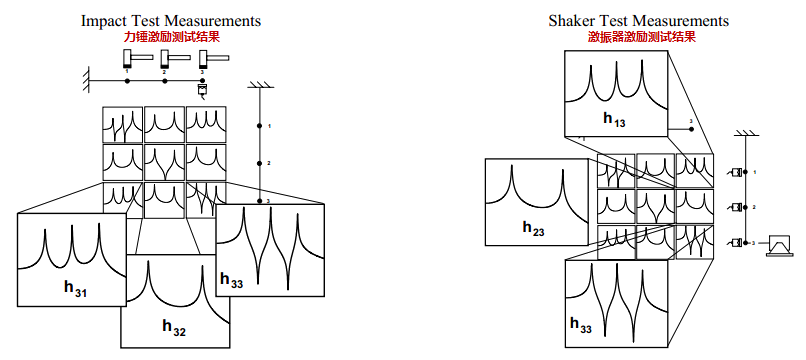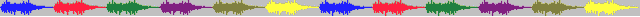# 3.模态空间98.06 — 模态试验用激振器激励还是用锤击激励之间有什么差别吗？

### 模态空间 – 在我们自己的小世界中     Pete Avitabile(著) KSI科尚仪器 董书伟（译）

#### 嗯… 问得好。答案是既有差别又没有差别。

$\left [ M \right ]\left \{ \ddot{x} \right \}+\left [ C \right ]\left \{ \dot{x} \right \}+\left [ K \right ]\left \{ x \right \}=\left \{ F\left ( t \right ) \right \}$

$\left [ \left [ M \right ]s^2+\left [ C \right ]s+\left [ K \right ] \right ]\left \{ X\left ( s \right ) \right \}=\left \{ F\left ( s \right ) \right \}\Rightarrow \left [ B\left ( s \right ) \right ]\left \{ X\left ( s \right ) \right \}=\left \{ F\left ( s \right ) \right \}$

$\left [ B\left ( s \right ) \right ]^{-1}=\left [ H\left ( s \right ) \right ]=\dfrac{Adj\left [ B\left ( s \right ) \right ]}{det\left [ B\left ( s \right ) \right ]}=\dfrac{\left [ A\left ( s \right ) \right ]}{det\left [ B\left ( s \right ) \right ]}$

$\left [ H\left ( s \right ) \right ]_{s=j \omega}=\left [ H\left ( j \omega \right ) \right ]=\displaystyle\sum_{k=1}^{m}\dfrac{\left [ A_{k} \right ]}{\left ( j \omega-p_{k} \right )}+\dfrac{\left [ A_{k}^{*} \right ]}{\left ( j \omega-p_{k}^{*} \right )}$

$h_{ij}\left ( j \omega \right )=\displaystyle\sum_{k=1}^{m}\dfrac{a_{ijk}}{\left ( j \omega-p_{k} \right )}+\dfrac{a_{ijk}^{*}}{\left ( j \omega-p_{k}^{*} \right )}$《振动：解析与试验模态分析》辛辛那提大学 Randall J. Allemang教授(著) KSI科尚仪器 董书伟(译)…